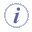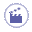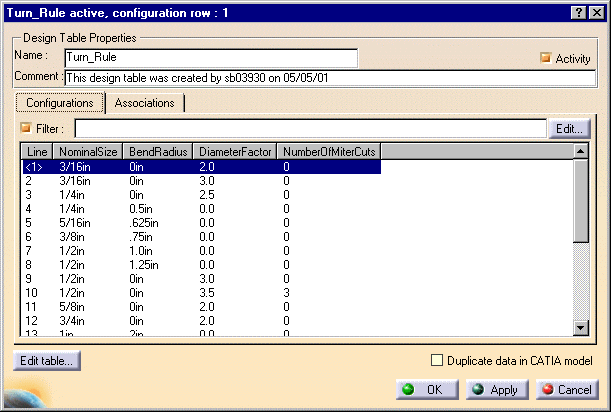# Turn RuleThis task explains the turn design rule.The turn design rule included with this application is used by the routing a run function. See Routing a Run for more information. It is used to establish the bend radius (also called turn radius) of a run if there is a value in the BendRadius column. You can enter more than one bend radius for a given nominal size, in which case the user has to select a bend radius when routing. If there is no value in the bend radius column then the bend radius is calculated by multiplying the value in the DiameterFactor column by the value in the Bend Diameter column of the Dimension design table. The bend radius value - whether it is from the turn rules table or calculated - displays in the turn radius field of the Run dialog box. If the value in the NumberofMiterCuts is other than 0 then your elbows will be mitered. The number of miters will equal the value shown in the column plus 1. If an input nominal size is not located in this table then an elbow is placed at turns. This is a specification-related design rule and the associated table is located in the directory ...\intel_a\Startup\EquipmentAndSystems\XXXXX\Specification where XXXXX is the application name, such as Tubing.1. The image below shows the turn rule design table for the Tubing Design product. Tables for other applications will have different entries but function in the manner described below.The NominalSize value is the input. Values in the BendRadius, DiameterFactor and NumberofMiterCuts columns are the output.